+6738639746 / 6732580443

Availability: In Stock
B\$48.31Full Refund if not receive order. See More Details### Secure Payment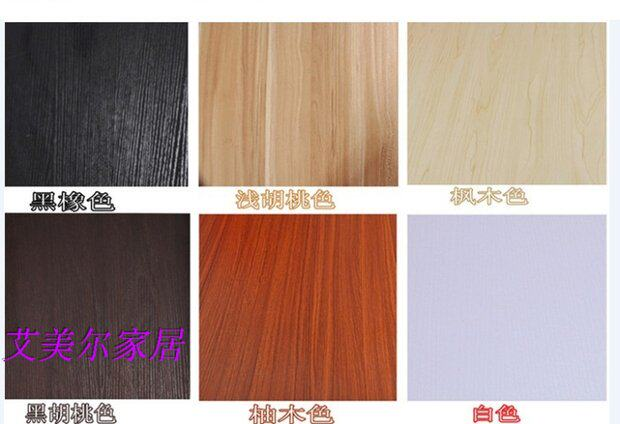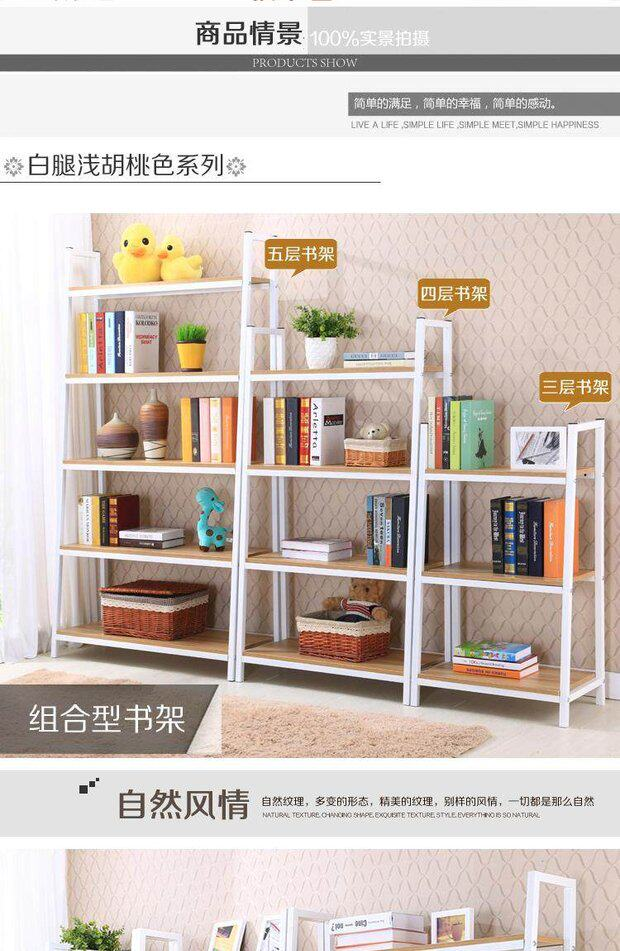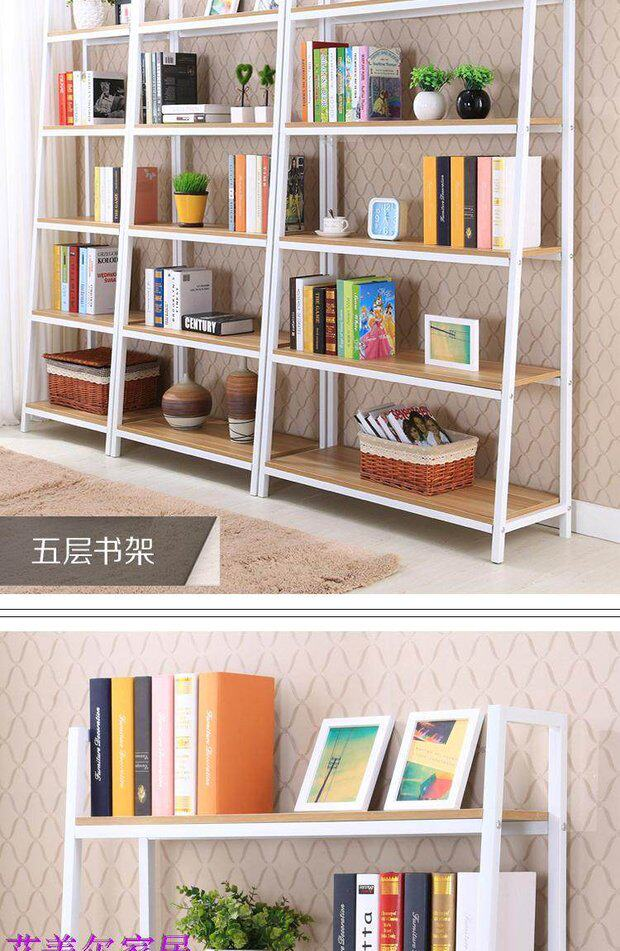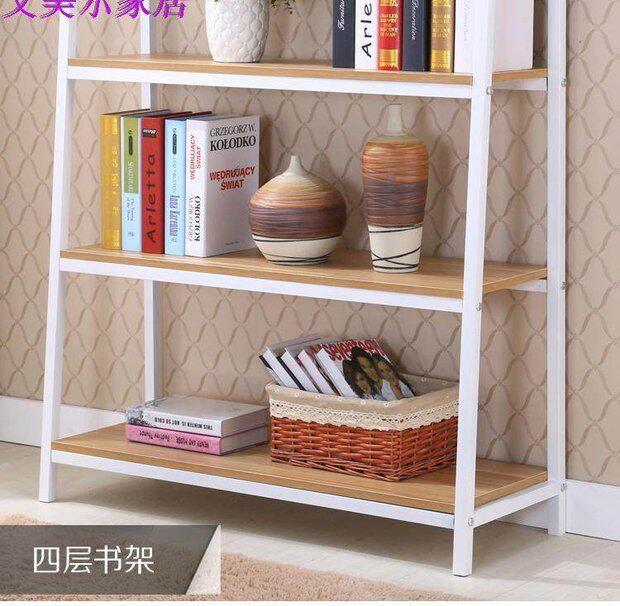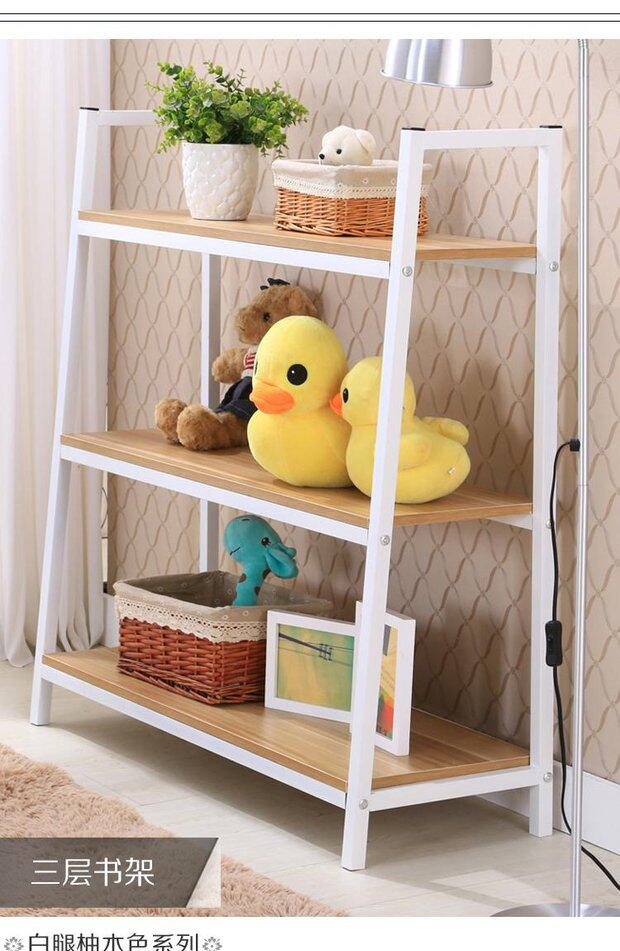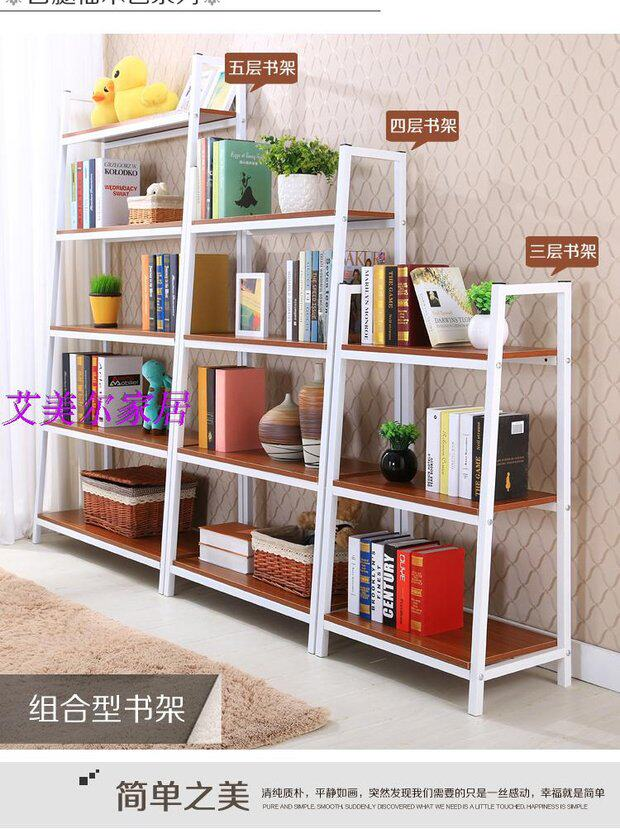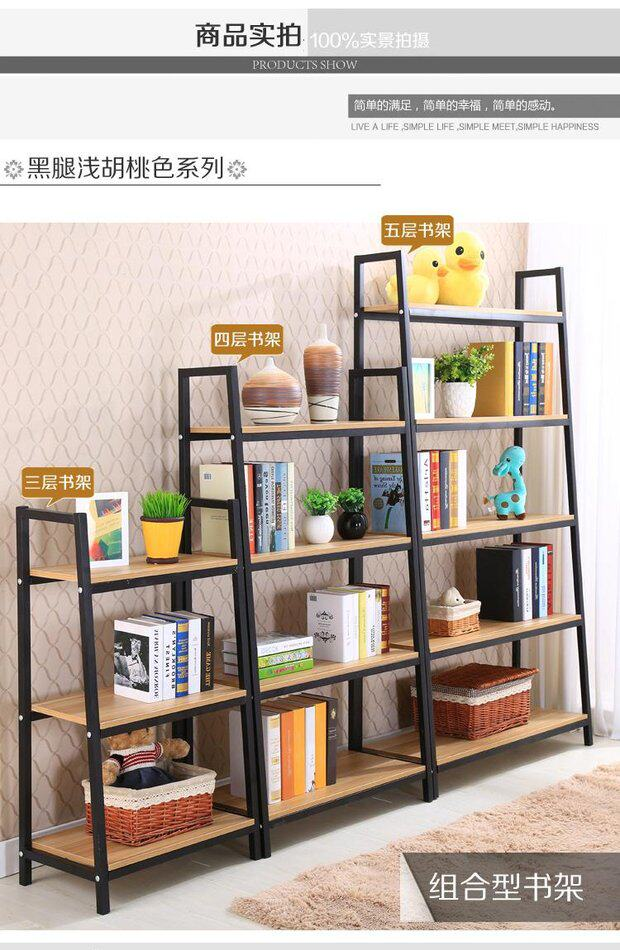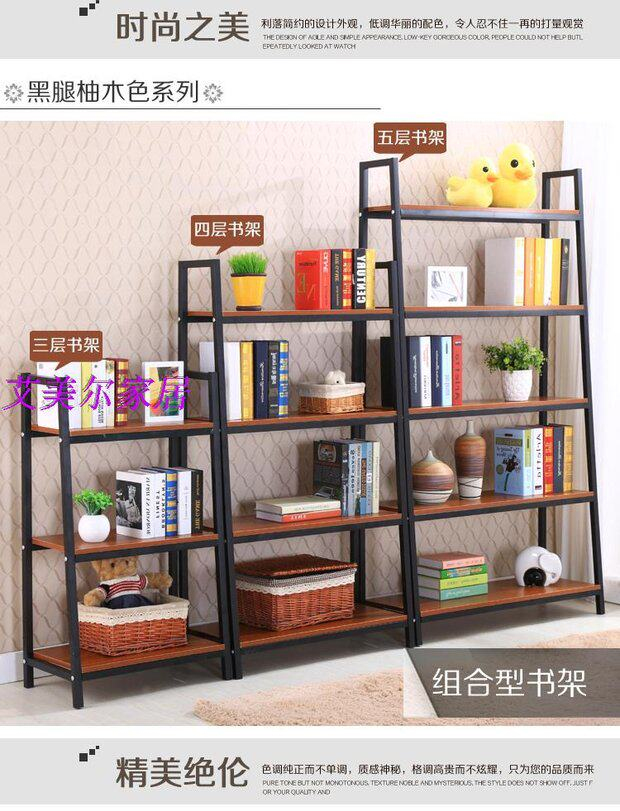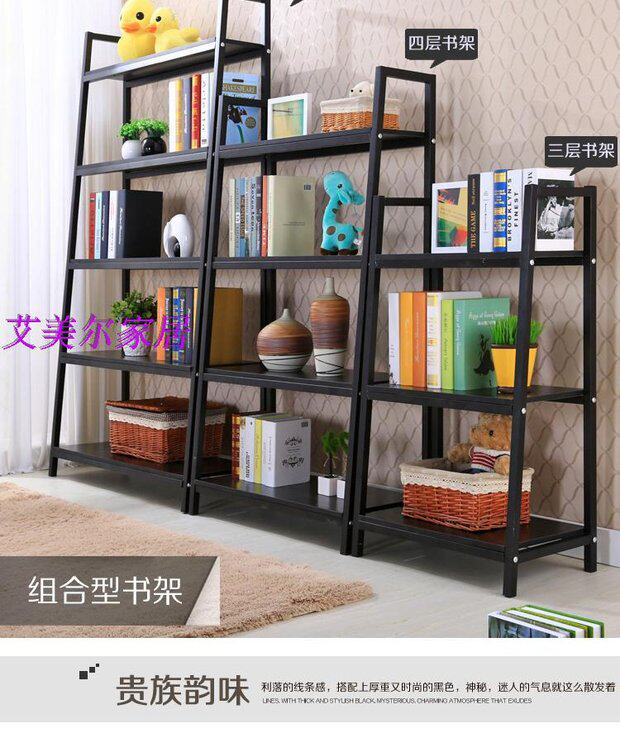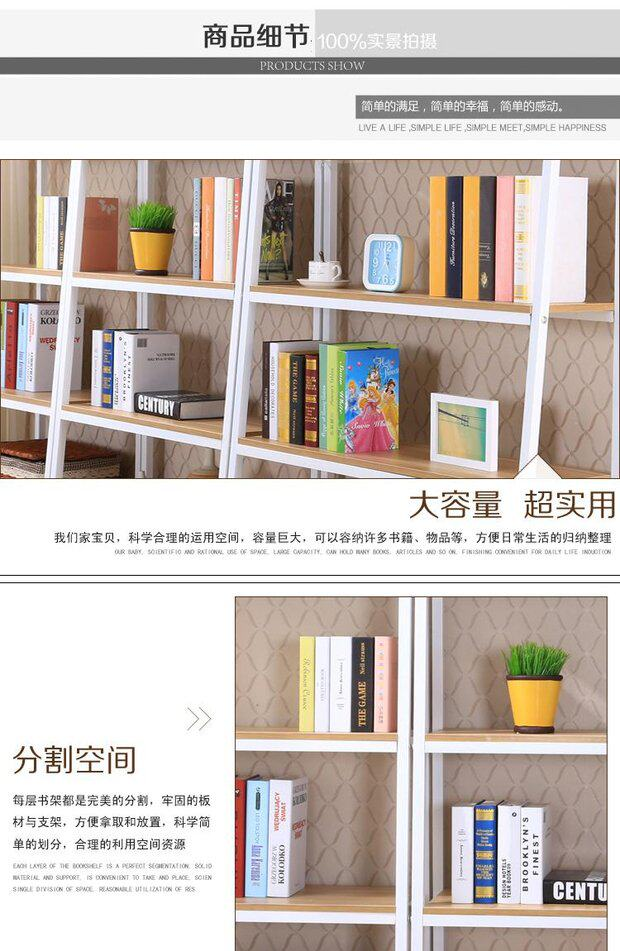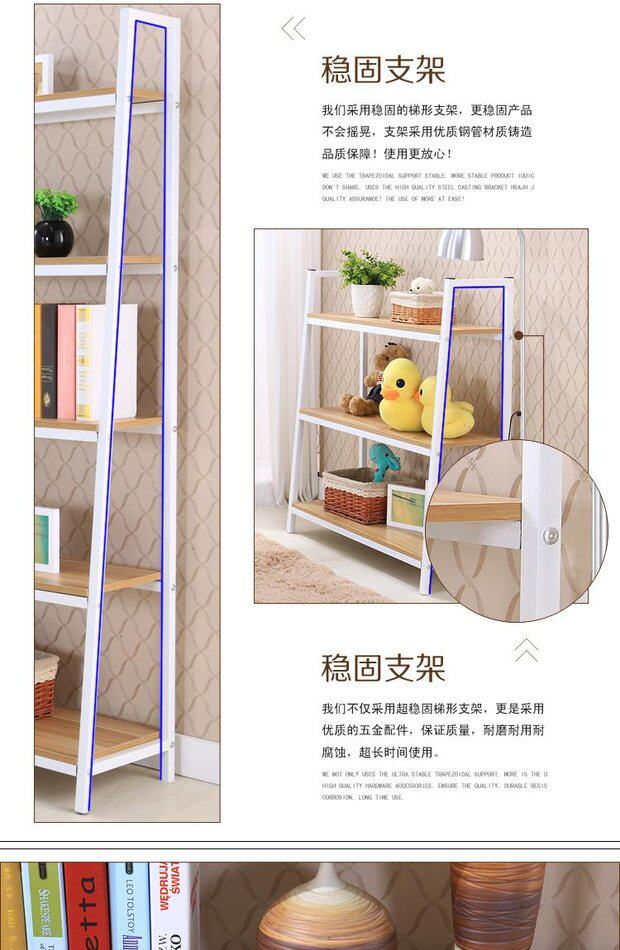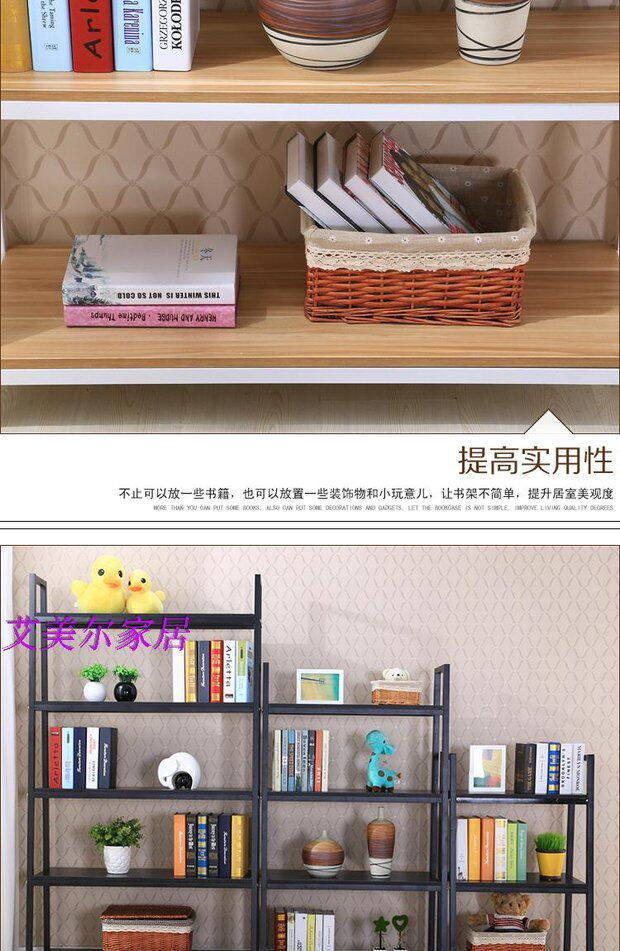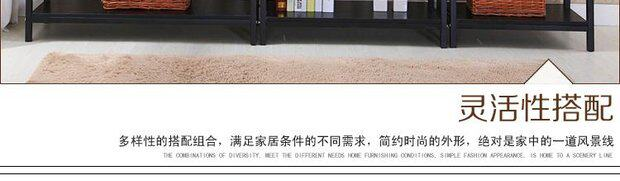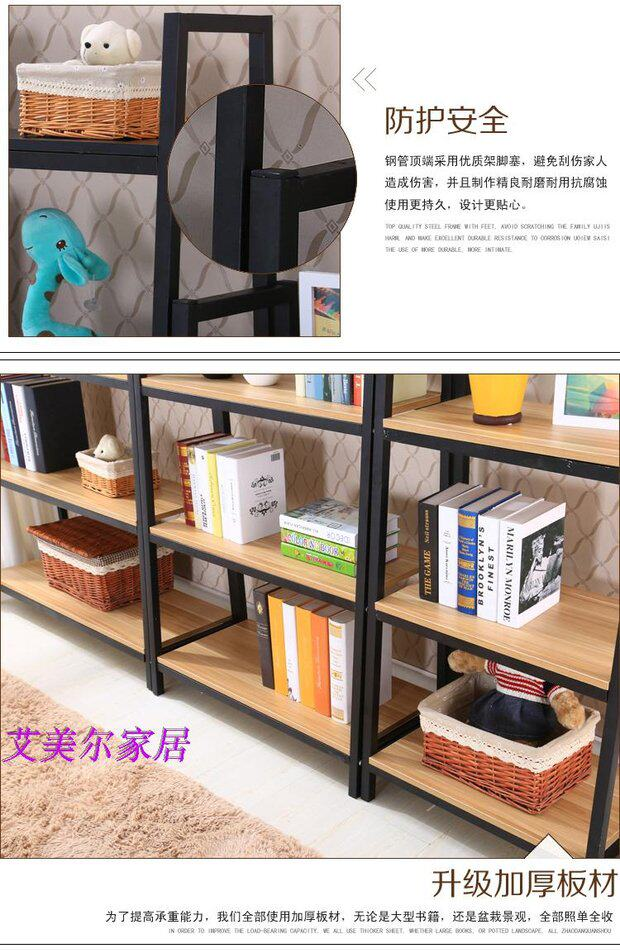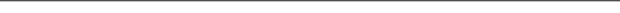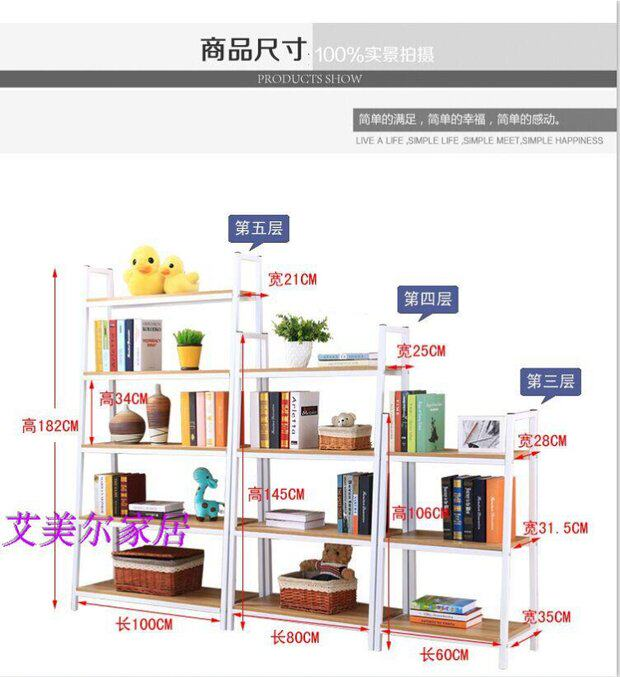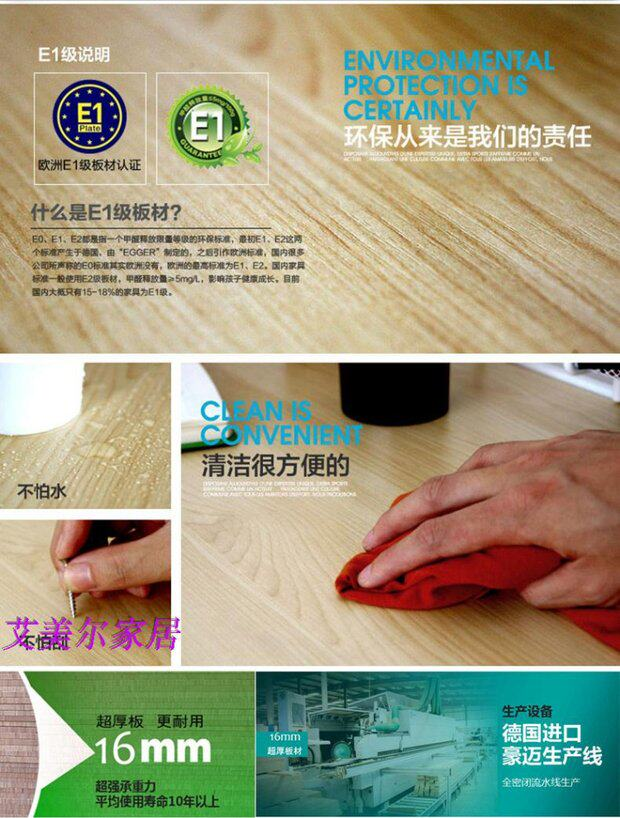#### Memory

• Amel home
• 0088
• Wood-based panel
• Particleboard/melamine board
• Jiangsu province
• The five floors are 1 meter long and 1.82 meters high,The five floors are 0.8 meters long and 1.82 meters high,The five floors are 1.5 meters long and 1.82 meters high,The length of the fourth floor is 1 meter and the height is 1.45 meters,The fourth floor is 0.8 meters long and 1.45 meters high,The fourth floor is 1.5 meters long and 1.45 meters high,The length of the three layers is 1 meter and the height is 1.06 meters,The three-story length is 0.8 meters and the height is 1.06 meters,The three floors are 1.5 meters long and 1.06 meters high,The five floors are 1.2 meters long and 1.82 meters high,The fourth floor is 1.2 meters long and 1.45 meters high,The three-story length is 1.2 meters and the height is 1.06 meters,The three-story length is 0.6 meters and the height is 1.06 meters,The five floors are 0.6 meters long and 1.82 meters high,The fourth floor is 0.6 meters long and 1.45 meters high
• Disassemble,Storage,Multifunction
• 115cm

Whatsapp Support